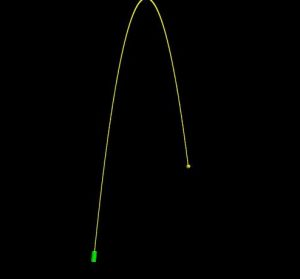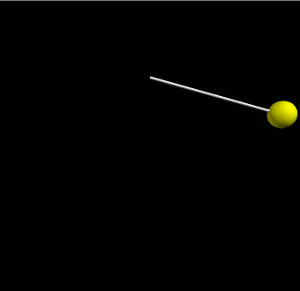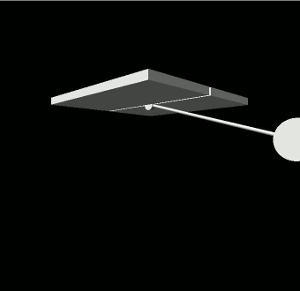# Cannonball in Visual Python

Just a very basic example of projectile motion in Visual Python. The cannon fires when the mouse is clicked in the window.```from visual import *

# Constants
g = 9.80            # (m/s^2)
v_0x = 5            # Initial speed in x-direction
v_0y = 50           # Initial speed in y-direction

# Set the display
scene = display(title='Cannonball',
x=0, y=0, width=700, height=700,
center=(0, 30, 0))

# Draw the cannon
location = [(0, 0, 0), (0.5, 5, 0)]
cannon = extrusion(pos=location, shape=outer-inner, color=color.green)

def position(t):
"""
Move the cannonball according to the equations of projectile motion.
"""
x = v_0x*t
y = v_0y * t - 0.5 * g * t ** 2
ball.pos = (x, y, 0)

# Shoot cannon when mouse is clicked and increment time
while True:
rate(1000)
if scene.mouse.clicked:
m = scene.mouse.getclick()
ball = sphere(pos=(0, 0, 0), radius=1, color=color.yellow, make_trail=True)
i = 0
flag = True
while flag:
rate(1000)
position(i)
i += 0.01
if ball.pos < 0:  # Stop before ball goes through the floor
flag = False
```

# Simple Pendulum in Visual Python

Below is the code for a simple pendulum in visual python.```from visual import sphere, color, rate, cylinder
import numpy as np

#
# Constants
#

g = 9.80            # (m/s^2)
L = 10              # Length of the pendulum
initialAngle = 1.3  # In radians

#
# Create the pendulum bob and rod
#
pend = sphere(pos=(L*np.sin(initialAngle), -L*np.cos(initialAngle), 0), radius=1, color=color.yellow)
rod = cylinder(pos=(0, 0, 0), axis=(pend.pos, pend.pos, 0), radius=0.1)

def position(time):
"""
Given time, t, this function moves the pendulum to its new position. We
use the equation:
theta(t) = theta_0*cos(sqrt(g/L)*t)
"""
theta = initialAngle*np.cos((g/L)**(1/2)*time)          # Angle of the pendulum
pend.pos = [L*np.sin(theta), -L * np.cos(theta), 0]     # Update position of bob
rod.axis = [pend.pos, pend.pos, 0]                # Update rod's position

# Increment time
i = 0
while True:
rate(100)
position(i)
i += 0.01

```

# Animated GIFs from Visual Python in Windows

One option for creating animated GIFs from your visual python program is add the pillow package that allows you to export images of your vpython window from within your program. You can then use GIMP to create an animation such as the one shown here.To use python’s image library, you can install the “pillow” package, and then use the line

```from PIL import ImageGrab
```

to import the necessary functionality. To take an image of your vpython display, add the following

```im = ImageGrab.grab((0, 0, 600, 600))
im.save('filename.jpg')
```

This code specifies that the image should be taken starting at the top left of your screen and extending down 600 pixels and right 600 pixels.

Once you have created a set of images, you can create the animated GIF using GIMP as follows:

1. Open GIMP and go to File > Open as Layers
2. Select all your images and click “Open”
3. Go to Filters > Animation > Playback to see your animation
4. Go to Filters > Animation > Optimize for GIF
5. When you are ready, go to File > Export As
6. Rename the image with the “.gif” extension.
7. Select the “animation” checkbox and the frame rate

The visual python code used to create the animation of the simple pendulum above, is shown below. Notice that I set the window size for convenience, and I placed the image-grabbing code in a loop, but only took an image every five iterations.

```from visual import *
import numpy as np
from PIL import ImageGrab

#
# Constants
#

g = 9.80            # (m/s^2)
L = 10              # Length of the pendulum
initialAngle = 1.3  # In radians

# Set the display window to make the image grabs easier
scene = display(title='Simple Pendulum',
x=0, y=0, width=700, height=700,
center=(0, -5, 0))

#
# Create the pendulum bob and rod
#
pend = sphere(pos=(L*np.sin(initialAngle), -L*np.cos(initialAngle), 0), radius=1, color=color.yellow)
rod = cylinder(pos=(0, 0, 0), axis=(pend.pos, pend.pos, 0), radius=0.1)

def position(time):
"""
Given time, t, this function moves the pendulum to its new position. We
use the equation:
theta(t) = theta_0*cos(sqrt(g/L)*t)
"""
theta = initialAngle*np.cos((g/L)**(1/2)*time)          # Angle of the pendulum
pend.pos = [L*np.sin(theta), -L * np.cos(theta), 0]     # Update position of bob
rod.axis = [pend.pos, pend.pos, 0]                # Update rod's position

# Increment time
i = 0
while True:
rate(100)
position(i)
i += 0.01
if (int(i * 100)) % 5 == 0:
im = ImageGrab.grab((10, 30, 690, 690))
im.save('Images/filename' + str(int(i*100)) + '.jpg')

```

# Fancy Simple Pendulum in Visual Python

Here is the visual python code for a “fancy” pendulum exhibiting simple harmonic motion. Clicking anywhere in the window will reset the pendulum to that angle.```
from __future__ import division, print_function
from visual import *
import numpy as np

# Set the constants
g = 9.80            # (m/s^2)
L = 10              # Length of the pendulum
initialAngle = 1.3  # In radians

# Make the pendulum bob, a small ceiling, a hinge, and the rod.
position = [L*np.sin(initialAngle), -L*np.cos(initialAngle), -0.15]
pend = cylinder(pos=position, axis=(0, 0, 0.3), radius=1)
box(pos=(0, 0.25, -2.6), length=10, height=0.5, width=5)
box(pos=(0, 0.25, 2.6), length=10, height=0.5, width=5)
cylinder(pos=(0, 0, -0.15), axis=(0, 0, 0.3), radius=0.4)
rod = cylinder(pos=(0, 0, 0), axis=(pend.pos, pend.pos, 0), radius=0.1)

def position(time):
"""
Given time, t, this function moves the pendulum to its new position. We
use the equation:
theta(t) = theta_0*cos(sqrt(g/L)*t)
"""
theta = initialAngle*np.cos((g/L)**(1/2)*time)          # Angle of the pendulum
pend.pos = [L*np.sin(theta), -L * np.cos(theta), -0.15] # Update position of bob
rod.axis = [pend.pos, pend.pos, 0]                # Update rod's position

i = 0
while True:
rate(100)
if scene.mouse.clicked:
m = scene.mouse.getclick()
angle = np.arctan2(m.pos, -m.pos)
pend.pos = [L * np.sin(angle), -L * np.cos(angle), -0.15]
rod.axis = [pend.pos, pend.pos, 0]
initialAngle = angle
else:
position(i)
i += 0.01

```